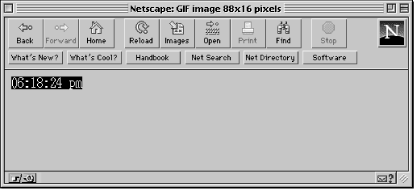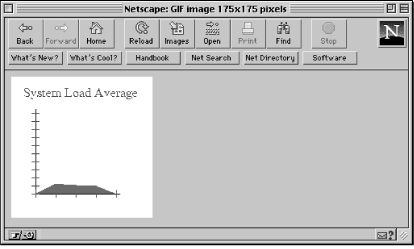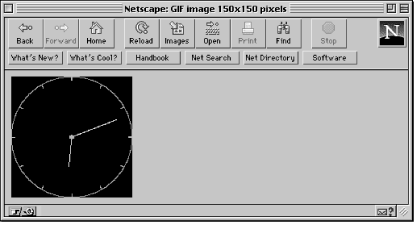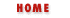#Chapter 6Hypermedia Documents## 6.2 CGI Examples with PostScript

PostScript is a language for laying out nicely designed pages with all kinds of fonts, pictures, and other things that HTML is not capable of displaying. PostScript on the screen often looks exactly like a page from a book or journal. The language is device independent, so it can be printed or displayed on any device that interprets it. Since most Web browsers don't handle PostScript code, it has to be run through an interpreter to produce an image that browsers can handle. Let's look at some examples that illustrate this concept.

### Digital Clock

In this example, we'll write PostScript code to create a virtual image of a digital clock displaying the current time. Since Web browsers can't display PostScript graphics, we will run this code through a PostScript interpreter, GNU GhostScript (freely available for many platforms), to create a GIF image which the browsers can easily handle. You should be conservative when creating dynamic graphics in this manner because GhostScript uses up a lot of system resources. If used wisely, however, these dynamic images can add a lot to your documents.

You can get GhostScript from the following location: http://www.phys.ufl.edu/ docs/goodies/unix/previewers/ghostscript.html.

Let's take a step-by-step look at this Perl script, which creates an image of a digital clock where the letters are red (Times Roman 14 point font) and the background is black.

```#!/usr/local/bin/perl
\$GS = "/usr/local/bin/gs";
\$| = 1;
print "Content-type: image/gif", "\n\n";
```

The first line of code just sets the \$GS variable to the path name of the GhostScript executable. You might need to change this to reflect the correct path on your system. Next, the \$| variable is set to 1, a Perl convention that makes the standard output unbuffered. Whenever you're outputting any type of graphics, it's better to unbuffer standard output, so Perl flushes the buffer after every print statement. Unfortunately, this degrades performance slightly because the buffer has to be flushed after every write. But it prevents occasional problems where the image data gets lost or corrupted.

And since we're creating a virtual GIF image, we need to output a MIME content type of image/gif.

```(\$seconds, \$minutes, \$hour) = localtime (time);
if (\$hour > 12) {
\$hour -= 12;
\$ampm = "pm";
} else {
\$ampm = "am";
}
if (\$hour == 0) {
\$hour = 12;
}
\$time = sprintf ("%02d:%02d:%02d %s", \$hour, \$minutes, \$seconds, \$ampm);
```

This code stores the current time as well as an "A.M." or "P.M." in the \$time variable.

```\$x = 80;
\$y = 15;
```

We set the image dimensions to 80x15 pixels. Horizontally, 80 pixels are enough to display our time string. And vertically, 15 pixels are sufficient to show a 14-point font.

```open (GS, "|\$GS -sDEVICE=gif8 -sOutputFile=- -q -g\${x}x\${y} - 2> /dev/null");
```

We use open to create a pipe (indicated by the "|" character) for output. This is the opposite of what we did in the previous example. Whatever data is written to the GS file handle is sent directly to GhostScript for execution (or interpretation); there is no need to store information in temporary files.

Several command-line options are used to GhostScript. The most important one is sDEVICE, which specifies the driver that GhostScript will use to create the output. Since we want a GIF image, we'll use the gif8 driver, which is packaged with the default GhostScript installation kit. (Warning: Some system administrators don't install all the default drivers, in which case the following program may not work.)

The -sOutputFile option with a value of "-" indicates that the output image data is to be written to standard output. The -q option turns off any informational messages output by GhostScript to standard output. This is very important because the text messages can corrupt the graphic data, as both are normally written to standard output stream. The -g option sets the dimensions for the output image.

The "-" instructs GhostScript to read PostScript data from standard input, because that's where our script is writing the PostScript code to. Finally, any error messages from GhostScript are discarded by redirecting the standard error to a null device, using the shell syntax 2>/dev/null.

```print GS <<End_of_PostScript_Code;
```

This print statement will write the PostScript code below to the file handle GS until it encounters the "End_of_PostScript_Code" string (another example of a "here" document).

```%!PS-Adobe-3.0 EPSF-3.0
%%BoundingBox: 0 0 \$x \$y
```

This is the start of the PostScript code. The first line, starting with %!PS-Adobe-3.0, is very important (it is much like the #! line used at the beginning of Perl scripts). It instructs GhostScript that the input consists of Encapsulated PostScript (EPS) commands. EPS was designed to allow various programs to share and manipulate a single PostScript graphic.

Since EPS was created to share graphic images, the BoundingBox statement in the second line specifies the position and size of the image that will be shared; in this case, the entire image. The EndComments statement ends the header section for the PostScript program.

Before we start examining the main part of our program, let's discuss how PostScript works. PostScript is different from many other programming languages in that it's stack based. What does that mean? If a command needs two arguments, these arguments must be placed "on the stack" before the command is executed. For example, if you want to add two numbers, say 5 and 7, you must place them on the stack first, and then invoke the add operator. The add operator adds the two numbers and places the result back on the stack. Here's the main part of the program:

```/Times-Roman findfont 14 scalefont setfont
```

The operand Times-Roman is first placed on the stack since the findfont operator expects one argument. The scalefont operator also needs one argument (14), and setfont needs two--the font name and the size, which are returned by the findfont and scalefont operators.

```/red   {1 0 0 setrgbcolor} def
/black {0 0 0 setrgbcolor} def
```

We proceed to define the two colors that we'll use in the image: red and black. The setrgbcolor operator needs three operands on the stack: the red, blue, and green indexes (ranging from 0--1) that comprise the color. Red is obtained by setting the red index to the maximum, and leaving the blue and green indices at zero. Black is obtained by setting all three indices to zero.

```black clippath fill
0 0 moveto
(\$time) red show
```

We use the fill command to fill the clipping region (which represents the entire drawing area) black, in essence creating a black background. The moveto command moves the "cursor" to the origin, which is the lower-left corner in PostScript. The show operator displays the string stored in the Perl variable \$time in red.

```showpage
```

Every PostScript program must contain the showpage operator, somewhere near the end. PostScript will not output the image until it sees this operator.

```End_of_PostScript_Code
close (GS);
exit(0);
```

The "End_of_PostScript_Code" string ends the print statement. The GS file handle is closed, and the program exits with a success status (zero).

Figure 6.1 shows how the output of this program will be rendered on a Web browser.

#### Figure 6.1: PostScript digital clockNow, how do you go about accessing this program? There are two ways. The first is to open the URL to this CGI program:

```http://your.machine/cgi-bin/digital.pl
```

Or, you can embed this image in another HTML document (either static or dynamic), like so:

```<IMG SRC="/cgi-bin/digital.pl">
```

This second method is very useful as you can include virtual graphics in a static or dynamic HTML document, as you will see in the following section.

### Inserting Multiple Dynamic Images

All of the programs we've discussed up to this point returned only one MIME content type. What if you want to create a dynamic HTML document with embedded virtual graphics, animations, and sound. Unfortunately, as of this writing, a CGI program cannot accomplish this task.

The closest we can get to having multiple heterogeneous information in a single document is embedding virtual images in a dynamic HTML document. Here is a simple example:

```#!/usr/local/bin/perl
\$digital_clock = "/cgi-bin/digital.pl";
print "Content-type: text/html", "\n\n";
print <<End_of_HTML;
.
. (some HTML code)
.
<IMG SRC="\$digital_clock">
.
. (some more HTML code)
.
End_of_HTML
exit(0);
```

When the server executes this CGI program, it returns a dynamic HTML document that consists of the virtual image created by the digital clock program discussed earlier. In other words, the server will execute the digital clock program, and place the output from it into the HTML document.

To reiterate, this technique works only when you are sending a combination of HTML and graphics. If you want to send other data formats concurrently, you'll have to wait until browsers support a special MIME content type that allows you to send more than one data format.

### Another Example: System Load Average

The digital clock example presented earlier in the chapter is a very simple example and doesn't use the full power of PostScript. Now, we'll look at an example that uses some of PostScript's powerful drawing operators to create a graph of the system load average:

```#!/usr/local/bin/perl
\$GS = "/usr/local/bin/gs";
\$| = 1;
print "Content-type: image/gif", "\n\n";
\$uptime = `/usr/ucb/uptime`;
(\$load_averages) = (\$uptime =~ /average: (.*)\$/);
```

In Perl, the "backtics" (`) allow you to execute a UNIX system command and store its output. In this case, we are storing the output from the uptime command into the variable \$uptime. The uptime command returns (among other things) three values representing the load average of the system in the last 5, 10, and 15 minutes (though this may differ among the various UNIX implementations).

I grab the output of uptime, strip it down to the load averages, and place the load averages into an array. Here is the output of a typical uptime command:

```  12:26AM  up 1 day,  17:35,  40 users,  load average: 3.55, 3.67, 3.53
```

A regular expression is used to retrieve data following the word "average:" up until the end of the line. This string, which contains the load averages separated by a comma and a space, is stored in the variable \$load_averages. The split operator splits (or separates) the data string on the comma and the space into three values that are stored in the array @loads.

```for (\$loop=0; \$loop <= 2; \$loop++) {
}
}
```

This loop iterates through the @loads array and reduces any load average over 10 to exactly 10. This makes it very easy for us to draw the graph. Otherwise, we need to calculate scaling coefficients and scale the graph accordingly.

```\$x = \$y = 175;
open (GS, "|\$GS -sDEVICE=gif8 -sOutputFile=- -q -g\${x}x\${y} - 2> /dev/null");
```

Through the \$x and \$y variables, the dimensions of the image are set to 175x175.

```print GS <<End_of_PostScript_Code;
%%BoundingBox: 0 0 \$x \$y
/black  {0 0 0 setrgbcolor} def
/red    {1 0 0 setrgbcolor} def
/blue   {0 0 1 setrgbcolor} def
/origin {0 dup} def
```

We use the setrgb operator to set the three colors (black, red, and blue) that we need to draw our image. The variable origin contains two zero values; the dup operator duplicates the top item on the stack. Note, the origin in PostScript is defined to be the lower-left corner of the image.

```15 150 moveto
/Times-Roman findfont 16 scalefont setfont
```

The moveto operator moves the "cursor" to point (15, 150). We use a blue Times-Roman 16 point for our title. The show operator displays the text.

```30 30 translate
```

translate is a very powerful operator. It moves (or translates, in mathematical terms) the coordinate axes from (0,0) to the point (30, 30). From here on, when we refer to point (0, 0), it will actually be point (30, 30) in the image. I did this translation to make the mathematics of drawing a figure easier.

```1 setlinewidth
origin moveto 105 0 rlineto black stroke
origin moveto 0 105 rlineto black stroke
```

Now we start to draw a figure showing the load average. We set the line width to be one pixel for all drawing operations. The rlineto operator draws two invisible lines from the origin--actually the point (30,30)--to the specified points. These lines are "painted" with the stroke operator. Since we are drawing a graph, these two lines represent the x and y axes in the graph.

Since a normal line extends from one point to the other, two coordinates are required to draw a line. But, in this case, we use the rlineto operator to specify coordinates relative to the current point (the origin).

```origin moveto
0 1 10 {
10 mul 5 neg exch moveto
10 0 rlineto blue stroke
} for
```

The loop shown above draws ten tick marks on the y axis. The for loop works the same as in any other language, with one minor exception. The loop variable (or counter) is placed on the top of the stack each time through the loop. In this case, the loop variable is multiplied by 10 on each iteration through the loop and placed on the stack. Then, a value of negative five is also placed on the stack. The two values on the stack (-5 and the counter multiplied by 10) represent the coordinates where a tick has to be drawn, and are swapped with the exch operator. From those coordinates, we draw a blue horizontal line that is 10 pixels in length.

To summarize, here is a step-by-step breakdown of the code we've just discussed:

• Move to the coordinates stored in the origin variable

• Execute the for loop 11 times (from 0 to 10 in increments of 1)

• Move to coordinates (-5, 10 x loop value)

• Draw a blue line from the above coordinates (-5, 10 x loop value) to (5, 10 x loop value) for a length of 10 pixels in the horizontal direction and repeat

• End of loop

Now, let's continue with the program.

```origin moveto
0 1 4 {
25 mul 5 neg moveto
0 10 rlineto blue stroke
} for
```

This procedure is nearly the same as the one discussed above, except that we are drawing vertical ticks on the x axis, where each tick mark is separated by 25 pixels (instead of 10), and is 10 pixels in length.

The code below draws five points: the origin, the three load average points, and a point on the x axis itself to "complete" the figure. Then we connect these points to create a filled region that represents the load average over time.

```newpath
origin moveto
```

The newpath operator establishes a new path. A path is used to create closed figures that can then be filled easily with the fill operator. Initially, we use the moveto operator to move to the origin. The load average is scaled by 10 and then used as the y coordinate. The x coordinate is simply incremented in steps of twenty--five-remember, each tick is separated by 25 pixels. Then, we draw a line using these two values. This procedure is repeated for all three load average values.

```100 0 lineto
closepath
red fill
showpage
End_of_PostScript_Code
```

A line is drawn from the last load average coordinate to the point directly on the x axis (100, 0). Finally, to close the figure, we draw a line from (100, 0) to the starting point of the path and fill it with red.

```close (GS);
exit(0);
```

This ends the PostScript section of our script. Back to Perl. The load average graph will look similar to the graph shown in Figure 6.2.

#### Figure 6.2: Graph of load averageAlthough it's possible to create graphs in PostScript (as we've just seen), it's much easier and quicker to use other utilities that were developed for the sole purpose of graphing numerical data. Several such utilities along with examples will be discussed later in this chapter.

### Final PostScript Example: Analog Clock

The final PostScript example we'll look at creates an analog clock using some of the more powerful PostScript operators. The image created by this program looks much like the one produced by the X Window System program xclock.

```#!/usr/local/bin/perl
\$GS = "/usr/local/bin/gs";
\$| = 1;
print "Content-type: image/gif", "\n\n";
(\$seconds, \$minutes, \$hour) = localtime (time);
\$x = \$y = 150;
open (GS, "|\$GS -sDEVICE=gif8 -sOutputFile=- -q -g\${x}x\${y} - 2> /dev/null");
print GS <<End_of_PostScript_Code;
%%BoundingBox: 0 0 \$x \$y
```

This initialization code is nearly the same in all of our PostScript examples so far, and should be familiar to you.

```/max_length     \$x def
/line_size      1.5 def
/marker         5 def
```

We start out by defining a lot of variables that are based on the values stored in the \$x and \$y variables. We do this so that if you increase the dimensions of the clock, all the objects of the clock (e.g., the minute and second hands) are scaled correctly. An important thing to note is that the x and y dimensions have to be equal for this automatic scaling to work properly.

The max_length variable sets the maximum length (or height, since this is a square clock) of the frame around the clock. The line width, used to draw the various objects, is stored in the line_size variable. The marker represents the length of the ticks (or markers) that represent the twelve hours on the clock.

```/origin         {0 dup} def
/center         {max_length 2 div} def
```

The origin contains the point (0, 0). Notice that whenever a variable declaration contains PostScript operators, we need to enclose the expression in braces. The center x (or y) coordinate of the clock (75, in this case) is stored in center. The radius of the circle that will encompass the entire drawing area is also 75, and is appropriately stored in the radius variable. The hour_segment contains the length of the line that will represent the hour value, which is half (or 50%) of the radius. The minute_segment contains the length of the minute hand, which is 80% of the radius. These are arbitrary values that make the clock look attractive.

```/red            {1 0 0 setrgbcolor} def
/green          {0 1 0 setrgbcolor} def
/blue           {0 0 1 setrgbcolor} def
/black          {0 0 0 setrgbcolor} def
```

We proceed to define four variables to hold the color values for red, green, blue, and black.

```/hour_angle {
\$hour \$minutes 60 div add 3 sub 30 mul
neg
} def
/minute_angle {
\$minutes \$seconds 60 div add 15 sub 6 mul
neg
} def
```

The angle of the hour and minute hands is calculated by the following formulas:

```hour angle = ((minutes / 60) + hour - 3) * 30
minute angle = ((seconds / 60) + minutes - 15) * 6
```

Try to understand these formulas. The derivation is pretty trivial if you know your trigonometry! Now, let's get to the real drawing routines.

```center dup translate
black clippath fill
line_size setlinewidth
origin radius 0 360 arc blue stroke
```

We use the translate operator to move the origin to the coordinate values stored in the variable center (in this case 75, 75). The fill operator fills the entire drawing area black. The setlinewidth operator sets the default line width for all drawing operations to 1.5 pixels. To finish the outline of the clock, we draw a blue circle. In PostScript terminology, we draw an arc from 0 to 360 degrees with the center at the origin and a radius of 75.

```gsave
1 1 12 {
pop
marker 0 rlineto red stroke
30 rotate
} for
grestore
```

Here is where the code gets a little complicated. We will discuss the gsave and grestore operators in a moment. Let's first look at the for loop, which draws the marks representing the 12 hours. Here is how it does it:

• Execute the for loop 12 times (from 1 to 12 in increments of 1)

• Remove the top value on the stack (or the loop counter) because we have no use for it!

• Move to the coordinate (radius - marker, 0)

• Draw a red line from (radius - marker, 0) to (marker, 0)

• Rotate the x and y axes by 30 degrees and repeat

• End of loop

The most important aspect of this loop is the rotation of the x and y axes, accomplished by the rotate command. This is one of the more powerful features of PostScript! By rotating the axes, all we have to do is draw straight lines, instead of calculating the coordinates for various angles. The gsave and grestore operators keep the rest of the drawing surface intact while the axes are being moved.

```origin moveto
hour_segment hour_angle cos mul
hour_segment hour_angle sin mul
lineto green stroke
origin moveto
minute_segment minute_angle cos mul
minute_segment minute_angle sin mul
lineto green stroke
origin line_size 2 mul 0 360 arc red fill
showpage
End_of_PostScript_Code
close (GS);
exit(0);
```

These statements are responsible for drawing the actual minute and second hands, as well as a small circle in the middle of the clock. The mathematical formulas to determine the hour angle are:

```hour (x coordinate) = cos (hour angle) * hour segment
hour (y coordinate) = sin (hour angle) * hour segment
```

The same theory is applied in calculating the angle for the second hand. Figure 6.3 shows how the analog clock will be rendered by a Web browser.

#### Figure 6.3: PostScript analog clockAs you can see from the PostScript examples that were presented, PostScript contains a lot of very powerful operators for creating and manipulating graphic images. However, you need to do a lot of work (and write complex code) to use PostScript effectively. In the next few sections, we will look at several other tools that will allow us to create dynamic images. These tools can't match the power of PostScript, but are easier to use and master.Creating Dynamic Home PagesThe gd Graphics Library